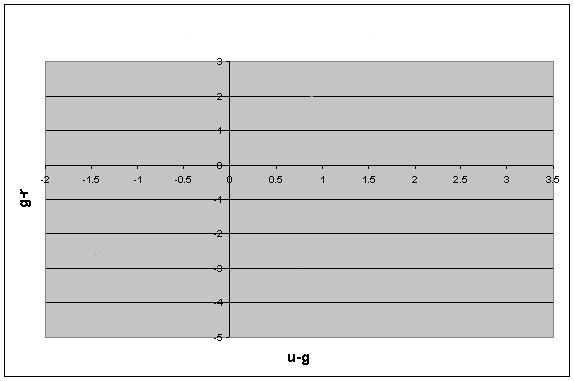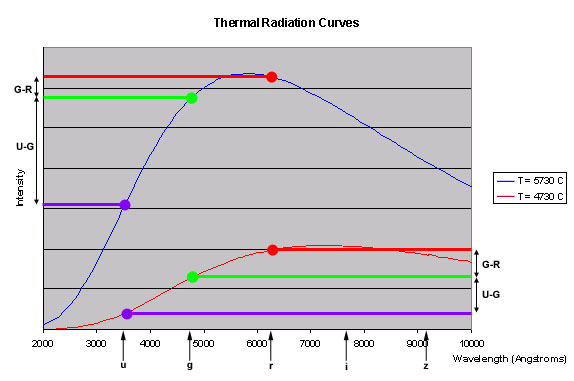To make a color-color diagram, find two colors of a single star; for example, g-r and u-g. Then, make a graph with u-g on the x-axis and g-r on the y-axis. The star you found will plot into a point on the color-color diagram. Find colors of several other stars and plot them on the same graph. The image at the right shows what a hypothetical color-color diagram might look like.

To understand how a thermal source should look on a color-color diagram, look at the thermal radiation curve below. The blue curve is for a star with T = 5730 C. The red curve is for a cooler star, at T = 4730 C. The graph shows snapshots from SDSS’s ultraviolet, green, and red filters.In both curves, the green-wavelength intensity is greater than the ultraviolet-wavelength intensity. That is, the green magnitude is less than the ultraviolet magnitude. Because magnitude decreases as the intensity of light emitted by a star increases, magnitude decreases moving up the y-axis on this graph. So u-g, which is the difference between the ultraviolet and green magnitudes, is positive for both curves, but it is less for the T = 4730 C curve. Similarly, g-r increases between the T = 5730 curve and the T = 4730 curve, although not as much as u-g.

Question 6. If you made a color-color diagram of these two stars, with u-g on the x-axis and g-r on the y-axis, what would you see?

Question 6 asks you what you would see if you made a color-color diagram for the two thermal sources graphed above. But what if you made a color-color diagram of many thermal sources? As you change the temperature, you change the thermal radiation curve. As the curve changes, the colors change. Because the filters are at fixed wavelengths, though, if one color (such as u-g) decreases, the next color (g-r) will increase. When you make a color-color diagram for thermal sources of different temperatures, you will get a straight line.

For the u-g/g-r color-color diagram, the line you get will be an extension of the line that connects the two points in Question 8. The slope of the line will be determined by the filters you use and the sources you examine.

Question 7. What would you get if you made a color-color diagram with g-r on the x-axis and r-i on the y-axis? With r-i on the x-axis and i-z on the y-axis?